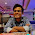Wednesday, July 30, 2014

Python Multiprocessing global variables

In the beginning, i want to say sorry, if this article will be "messy"...

One day i've noticed, that threading module in python does not working as should be.
Some times it was much slower than in sequential process. Then i learned about GIL (Global Interpreter Lock).

My teacher advised me to use Multiprocessing module.
Fine. It is very simple, just copy/replace:

That's all! It will work. But how?

In 'Threading' module, threads have shared memory, Threads can manipulate global variables of main thread, instead of multiprocessing module, that runs another subprocess in memory and it does not have shared memory like threading.

For example:
 ```#Threading Example from threading import Thread #defining a global variable mylist = [] def somefunc(a): global mylist mylist.append(a) def main() for i in range(100): t = Thread(target=somefunc,args=(i,)) t.start() t.join() ``` ```#Multiprocessing Example from multiprocessing import Process #defining a global variable mylist = [] def somefunc(a): global mylist mylist.append(a) def main() for i in range(100): t = Process(target=somefunc,args=(i,)) t.start() t.join() ```
In Threading Example, 'somefunc()' will append to the global 'mylist' variable, instead of Multiprocessing will be empty as it was before.

Solution for this issue came Manager objects of Multiprocessing module.
```from multiprocessing import Process,Manager

mylist = Manager.list()

def somefunc(a):
mylist.append(a)

def main()
for i in range(100):
t = Process(target=somefunc,args=(i,))
t.start()
t.join()

```

In one hand, this will help, but in another you will get headache. Because, if you add for example KeyboardInterrupt (^C) support, you will get nothing. Manager object will be empty. OK. Maybe my knowledge is not so good, but i've found another solution to manage variables: Callback function.

But before that, let's add some process control. I want to control how many processes running simultaneously:
```from multiprocessing import Pool,cpu_count,active_children

mylist = Manager.list()

def somefunc(a):
mylist.append(a)

def main()

#creating pool of worker processes, for 4 Cores will be 40 processes.
pool = Pool(processes=cpu_count()*10)
for i in range(100):

#start processes asynchronous, without waiting until process ends.
pool.apply_async(somefunc, (i,))
pool.close()

#waiting for results of ALL processes
while len(active_children()) > 1:
sleep(0.5)
pool.join()
```
In this example, there will be no more than 40 processes running at the same time.

Now, will add the Callback function:
```from multiprocessing import Pool,cpu_count,active_children

mylist = []

def somefunc(a):
a += 1
return a

def main()
def cb(data):
if data:
global mylist
mylist.append(data)

pool = Pool(processes=cpu_count()*10)
for i in range(100):
pool.apply_async(somefunc, (i,), callback=cb)
pool.close()
while len(active_children()) > 1:
sleep(0.5)
pool.join()
```

Every process will return some data to main process, then will be called callback function, that will manipulate with the data.
For me, callback function is much more easy for use and understand...

Next time will try to tell about successful implementation of KeyboardInterrupt ^C into multiprocessing script. It's another issue.

1.after one year... ~1000 views of this post, there are too many errors and no one commented...

2.Thanks for you sharing. you are right, there are many errors in your codes, I doubt if you have test them on machine~
These day I have meet same trouble, Have you solve problems above?
I think I need your help...

3.4.when i start a process in the code, i that the global section of the code is executed for each process. Is there a way to eliminate this ? For example

# global section
print "i am in global section"

if __name__ == "__main__":
myprocess = multiprocessing.Process()
myprocess.start()

output:
I am in global data
I am in global data

Hope my question is clear.

1.1. Create a function, and call it from 'if __name__ == '__main__'. And not at start of a script.
2. If you're making multiprocessing working on windows, there might be some other problems. Above code is useful for linux. I'm not coding for windows sorry :)

5.mylist = Manager.list() shoule be mylist = Manager().list()

6.instead of interrupt you can use Process.terminate(), and then wait using Process.join() for clean exit.